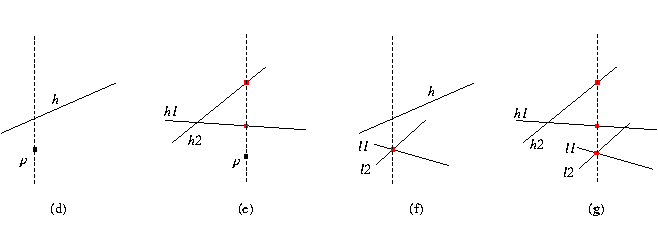## CGAL::compare_y_at_x

Comparison_result compare_y_at_x ( Point_2<Kernel> p, Line_2<Kernel> h)
compares the y-coordinates of p and the vertical projection of p on h, see (d) in the figure below.
Precondition: h is not vertical.

Comparison_result
 compare_y_at_x ( Point_2 p, Line_2 h1, Line_2 h2)
compares the y-coordinates of the vertical projection of p on h1 and on h2, see (e) in the figure below.
Precondition: h1 and h2 are not vertical.

Comparison_result
 compare_y_at_x ( Line_2 l1, Line_2 l2, Line_2 h)
Let p be the intersection of lines l1 and l2. This function compares the y-coordinates of p and the vertical projection of p on h, see (f) in the figure below.
Precondition: l1, l2 intersect and h is not vertical.

Comparison_result
 compare_y_at_x ( Line_2 l1, Line_2 l2, Line_2 h1, Line_2 h2)
Let p be the intersection of lines l1 and l2. This function compares the y-coordinates of the vertical projection of p on h1 and on h2, see (g) in the figure below.
Precondition: l1 and l2 intersect; h1 and h2 are not vertical.

Comparison_result
 compare_y_at_x ( Point_2 p, Segment_2 s)
compares the y-coordinates of p and the vertical projection of p on s. If s is vertical, then return EQUAL when p lies on s, SMALLER when p lies under s, and LARGER otherwise.
Precondition: p is within the x range of s.

Comparison_result
 compare_y_at_x ( Point_2 p, Segment_2 s1, Segment_2 s2)
compares the y-coordinates of the vertical projection of p on s1 and on s2. If s1 or s2 is vertical, then return EQUAL if they intersect, otherwise return SMALLER if s1 lies below s2, and return LARGER otherwise.
Precondition: p is within the x range of s1 and s2.